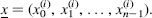## 11.5 KNAPSACK ENCIPHERMENT AND DECIPHERMENT OF ASCII-PLAINTEXT

Knapsack encipherment derives a μ-bit ciphertext integer B(i) from each plaintext (0,1}-vectorThen Internet standard [Linn, 1989] specifies the translation from ASCII text for Merkle–Hellman encipherment. I use a similar coding translation scheme illustrated in Example 11.11, which follows.

### 11.5.1 Knapsack Encipherment of ASCII-Plaintext

Plaintext: x(0) x(1)x(N−1) (ASCII characters)

Kuapsack Public Parameter. a = (a0, a1, …, an−1)

Ciphertext. y = (y(0), y(1), …, y(M − 1))-vectors.

E1. Each of the N ASCII plaintext characters x(i) in first coded into the 7-bit binary representation of its ordinal position in the ASCII character setE2. The vectors {x(i)} are concatenated to form the binary plaintextE3. The binary plaintext z is divided into equal length blocks of n bits, padding z on the right by 0's if necessary. By this processblocks of n bits are obtained

E4. For each bit-vector (z(i)), the integer is computed; ...

Get Computer Security and Cryptography now with the O’Reilly learning platform.

O’Reilly members experience live online training, plus books, videos, and digital content from nearly 200 publishers.